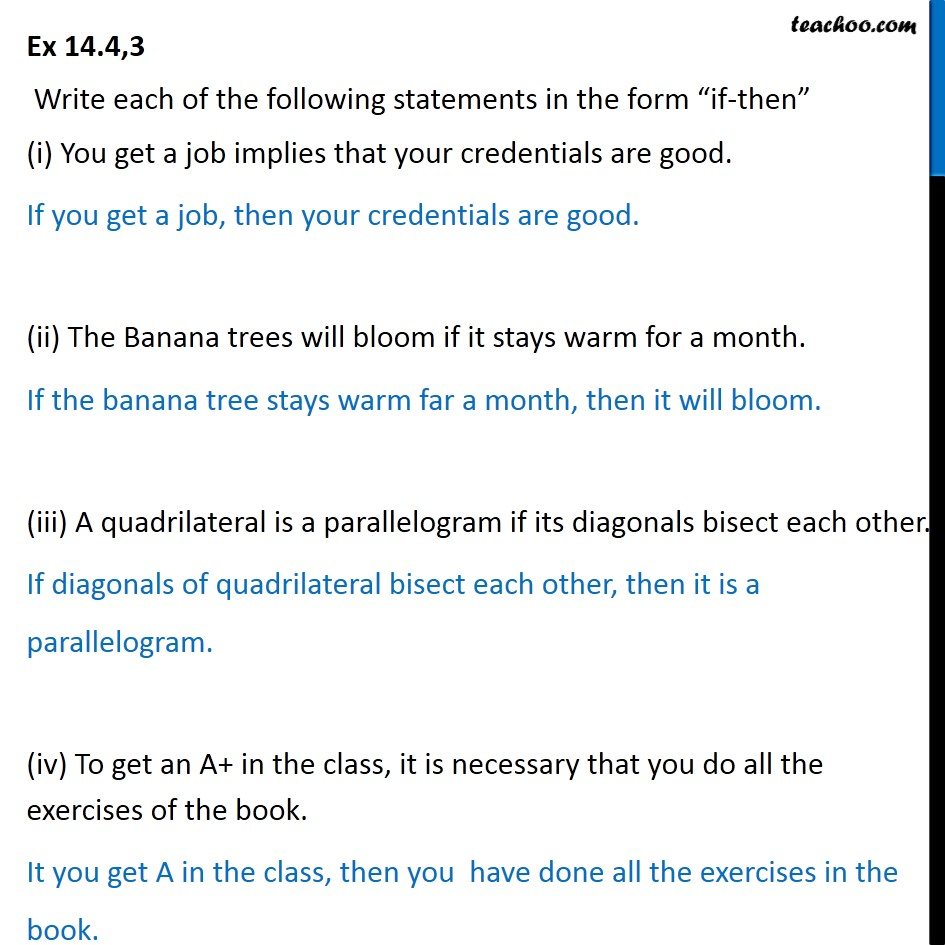1. Chapter 14 Class 11 Mathematical Reasoning
2. Concept wise
3. If then

Transcript

Ex 14.4,3 Write each of the following statements in the form if-then (i) You get a job implies that your credentials are good. If you get a job, then your credentials are good. (ii) The Banana trees will bloom if it stays warm for a month. If the banana tree stays warm far a month, then it will bloom. (iii) A quadrilateral is a parallelogram if its diagonals bisect each other. If diagonals of quadrilateral bisect each other, then it is a parallelogram. (iv) To get an A+ in the class, it is necessary that you do all the exercises of the book. It you get A in the class, then you have done all the exercises in the book.

If then